# Introduction

In this article, we will develop an application using Python. The standard library tkinter is used as the GUI library. If you have some understanding of python by reading this article, you can learn how to use tkinter to some extent.

# What is Tkinter

It is one of the standard Python libraries. A library for building GUI applications. A GUI library featuring a simple grammar and quick startup.

# Creating a window

First, let's create the basic window.

``````import tkinter as tk

if __name__ == "__main__":
root = tk.Tk()
root.mainloop()
``````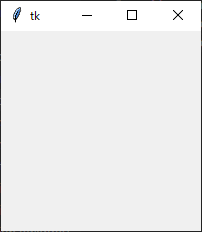# Creating a screen

Next, we will create a class that inherits the Frame class in order to write with object orientation in mind. Create the necessary parts (widgets) in create_widgets (), arrange them, and create the screen.

``````
import tkinter as tk

class Application(tk.Frame):
def __init__(self, master):
super().__init__(master)
self.pack()

master.geometry("300x200")
master.title("Typing game!")

self.create_widgets()

#Widget generation and placement
def create_widgets(self):
self.q_label = tk.Label(self, text="theme:", font=("",20))
self.q_label.grid(row=0, column=0)
self.q_label2 = tk.Label(self, text="tkinter", width=5, anchor="w", font=("",20))
self.q_label2.grid(row=0, column=1)
self.ans_label.grid(row=1, column=0)
self.ans_label2 = tk.Label(self, text="tkinter", width=5, anchor="w", font=("",20))
self.ans_label2.grid(row=1, column=1)
self.result_label = tk.Label(self, text="Right / wrong label", font=("",20))
self.result_label.grid(row=2, column=0, columnspan=2)

if __name__ == "__main__":
root = tk.Tk()
Application(master=root)
root.mainloop()
``````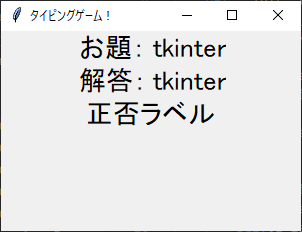# Keystroke event processing settings

Next, we will create the key input processing part. First, implement the process of adding a value to the answer column when a character is entered.

First, leave the initial value of the answer column as an empty string.

``````self.ans_label2 = tk.Label(self, text="", width=5, anchor="w", font=("",20))
``````

Next, create a method for event processing at the time of key input. The entered key information is stored in event.keysym. For example, if you press the A key, the information "a" is stored.

``````#Event processing at the time of key input
def type_event(self, event):
self.ans_label2["text"] += event.keysym
``````

Use bind () to link the last created event process to the Tk class instance.

``````#Implemented key event handling for Tk instances
self.master.bind("<KeyPress>", self.click_event)
``````

The summary up to this point is as follows.

``````import tkinter as tk

class Application(tk.Frame):
def __init__(self, master):
super().__init__(master)
self.pack()

master.geometry("300x200")
master.title("Typing game!")

self.create_widgets()

#Implemented key event handling for Tk instances
self.master.bind("<KeyPress>", self.type_event)

#Widget generation and placement
def create_widgets(self):
self.q_label = tk.Label(self, text="theme:", font=("",20))
self.q_label.grid(row=0, column=0)
self.q_label2 = tk.Label(self, text="tkinter", width=5, anchor="w", font=("",20))
self.q_label2.grid(row=0, column=1)
self.ans_label.grid(row=1, column=0)
self.ans_label2 = tk.Label(self, text="", width=5, anchor="w", font=("",20))
self.ans_label2.grid(row=1, column=1)
self.result_label = tk.Label(self, text="Right / wrong label", font=("",20))
self.result_label.grid(row=2, column=0, columnspan=2)

#Event processing at the time of key input
def type_event(self, event):
self.ans_label2["text"] += event.keysym

if __name__ == "__main__":
root = tk.Tk()
Application(master=root)
root.mainloop()
``````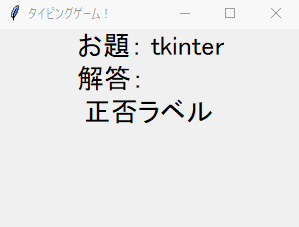# Implementation of answer matching process

This time, we will create it with the specification that if you press the "Enter key", the correct answer will be judged. The input key is judged using the value of event.keysym. When you press the "Enter key", "Return" is stored in event.keysym, so if it is judged. By the way, "keysym" is an abbreviation for "key symbol".

``````    #Event processing at the time of key input
def type_event(self, event):
#If the input value is Enter, answer together
if event.keysym == "Return":
if self.q_label2["text"] == self.ans_label2["text"]:
else:
self.result_label.configure(text="Sorry!", fg="blue")

self.ans_label2.configure(text="")

else:
#If the input value is other than Enter, add it to the label as a character input.
self.ans_label2["text"] += event.keysym
``````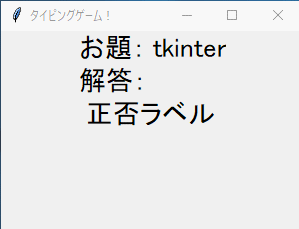# Implementation of continuous question function

Implement the function to proceed to the next problem after the pass / fail judgment.

First, create a list of problems.

``````
QUESTION = ["tkinter", "geometry", "widgets", "messagebox", "configure",
"label", "column", "rowspan", "grid", "init"]
``````

Next, prepare a variable for index that manages the number of problems in the constructor.

``````
#Problem number index
self.index = 0
``````

Finally, add the process of rewriting the label value to the next problem in the event process.

``````#Ask the next question
self.index += 1
self.q_label2.configure(text=QUESTION[self.index])
``````

The summary up to this point is as follows.

``````import tkinter as tk

QUESTION = ["tkinter", "geometry", "widgets", "messagebox", "configure",
"label", "column", "rowspan", "grid", "init"]

class Application(tk.Frame):
def __init__(self, master):
super().__init__(master)
self.pack()

master.geometry("300x200")
master.title("Typing game!")

#Problem number index
self.index = 0

self.create_widgets()

#Implemented key event handling for Tk instances
self.master.bind("<KeyPress>", self.type_event)

#Widget generation and placement
def create_widgets(self):
self.q_label = tk.Label(self, text="theme:", font=("",20))
self.q_label.grid(row=0, column=0)
self.q_label2 = tk.Label(self, text=QUESTION[self.index], width=10, anchor="w", font=("",20))
self.q_label2.grid(row=0, column=1)
self.ans_label.grid(row=1, column=0)
self.ans_label2 = tk.Label(self, text="", width=10, anchor="w", font=("",20))
self.ans_label2.grid(row=1, column=1)
self.result_label = tk.Label(self, text="Right / wrong label", font=("",20))
self.result_label.grid(row=2, column=0, columnspan=2)

#Event processing at the time of key input
def type_event(self, event):
#If the input value is Enter, answer together
if event.keysym == "Return":
if self.q_label2["text"] == self.ans_label2["text"]:
else:
self.result_label.configure(text="Sorry!", fg="blue")

self.ans_label2.configure(text="")

self.index += 1
self.q_label2.configure(text=QUESTION[self.index])

else:
#If the input value is other than Enter, add it to the label as a character input.
self.ans_label2["text"] += event.keysym

if __name__ == "__main__":
root = tk.Tk()
Application(master=root)
root.mainloop()

``````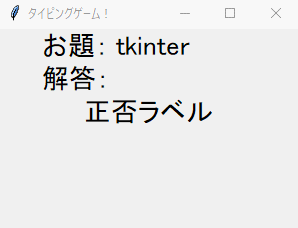# Implementation of backspace function

Next, we will implement the backspace function. Implement by adding a branch when event.keysym is "BackSpace".

``````    #Event processing at the time of key input
def type_event(self, event):
#If the input value is Enter, answer together
if event.keysym == "Return":
if self.q_label2["text"] == self.ans_label2["text"]:
else:
self.result_label.configure(text="Sorry!", fg="blue")

self.ans_label2.configure(text="")

self.index += 1
self.q_label2.configure(text=QUESTION[self.index])
elif event.keysym == "BackSpace":
text = self.ans_label2["text"]
self.ans_label2["text"] = text[:-1]
else:
#If the input value is other than Enter, add it to the label as a character input.
self.ans_label2["text"] += event.keysym
``````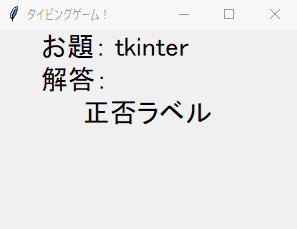# Implementation of result display function

Implement the function to display the result after answering to the end. The results are displayed using a pop-up window. Also, this time, we will make the specification so that the application will be forcibly terminated at the same time as closing the pop-up.

To implement the above process, first import two libraries.

``````from tkinter import messagebox
import sys
``````

Also, prepare a variable to count the number of correct answers. Let's initialize this in the constructor.

``````#For counting the number of correct answers
self.correct_cnt = 0
``````

Finally, go to the next problem in the event process to determine if you have reached the end of the problem and call the process to display the result pop-up (message box). sys.exit (0) is a method to kill the program.

``````#Ask the next question
self.index += 1
if self.index == len(QUESTION):
self.q_label2.configure(text="Finished!")
messagebox.showinfo("result", f"Your score is{self.correct_cnt}/{self.index}The question is correct.")
sys.exit(0)
self.q_label2.configure(text=QUESTION[self.index])
``````

The summary up to this point is as follows.

``````import tkinter as tk
from tkinter import messagebox
import sys

QUESTION = ["tkinter", "geometry", "widgets", "messagebox", "configure",
"label", "column", "rowspan", "grid", "init"]

class Application(tk.Frame):
def __init__(self, master):
super().__init__(master)
self.pack()

master.geometry("300x200")
master.title("Typing game!")

#Problem number index
self.index = 0

#For counting the number of correct answers
self.correct_cnt = 0

self.create_widgets()

#Implemented key event handling for Tk instances
self.master.bind("<KeyPress>", self.type_event)

#Widget generation and placement
def create_widgets(self):
self.q_label = tk.Label(self, text="theme:", font=("",20))
self.q_label.grid(row=0, column=0)
self.q_label2 = tk.Label(self, text=QUESTION[self.index], width=10, anchor="w", font=("",20))
self.q_label2.grid(row=0, column=1)
self.ans_label.grid(row=1, column=0)
self.ans_label2 = tk.Label(self, text="", width=10, anchor="w", font=("",20))
self.ans_label2.grid(row=1, column=1)
self.result_label = tk.Label(self, text="", font=("",20))
self.result_label.grid(row=2, column=0, columnspan=2)

#Event processing at the time of key input
def type_event(self, event):
#If the input value is Enter, answer together
if event.keysym == "Return":
if self.q_label2["text"] == self.ans_label2["text"]:
self.correct_cnt += 1
else:
self.result_label.configure(text="Sorry!", fg="blue")

self.ans_label2.configure(text="")

self.index += 1
if self.index == len(QUESTION):
self.q_label2.configure(text="Finished!")
messagebox.showinfo("result", f"Your score is{self.correct_cnt}/{self.index}The question is correct.")
sys.exit(0)
self.q_label2.configure(text=QUESTION[self.index])

elif event.keysym == "BackSpace":
text = self.ans_label2["text"]
self.ans_label2["text"] = text[:-1]

else:
#If the input value is other than Enter, add it to the label as a character input.
self.ans_label2["text"] += event.keysym

if __name__ == "__main__":
root = tk.Tk()
Application(master=root)
root.mainloop()
``````

I think that the basic functions have been implemented. The execution result is as follows.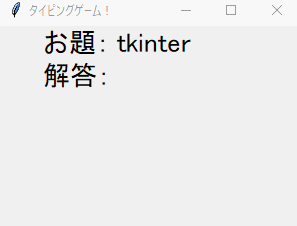As a bonus, if you can measure the time in real time using multi-thread processing, it will be as follows.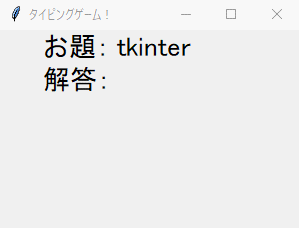The source code is as follows.

``````import tkinter as tk
from tkinter import messagebox
import sys
import time

QUESTION = ["tkinter", "geometry", "widgets", "messagebox", "configure",
"label", "column", "rowspan", "grid", "init"]

class Application(tk.Frame):
def __init__(self, master):
super().__init__(master)
self.pack()

master.geometry("300x200")
master.title("Typing game!")

#Problem number index
self.index = 0

#For counting the number of correct answers
self.correct_cnt = 0

self.create_widgets()

t.start()

#Implemented key event handling for Tk instances
self.master.bind("<KeyPress>", self.type_event)

#Widget generation and placement
def create_widgets(self):
self.q_label = tk.Label(self, text="theme:", font=("",20))
self.q_label.grid(row=0, column=0)
self.q_label2 = tk.Label(self, text=QUESTION[self.index], width=10, anchor="w", font=("",20))
self.q_label2.grid(row=0, column=1)
self.ans_label.grid(row=1, column=0)
self.ans_label2 = tk.Label(self, text="", width=10, anchor="w", font=("",20))
self.ans_label2.grid(row=1, column=1)
self.result_label = tk.Label(self, text="", font=("",20))
self.result_label.grid(row=2, column=0, columnspan=2)

# #Label for time measurement
self.time_label = tk.Label(self, text="", font=("",20))
self.time_label.grid(row=3, column=0, columnspan=2)

self.flg2 = True

#Event processing at the time of key input
def type_event(self, event):
#If the input value is Enter, answer together
if event.keysym == "Return":
if self.q_label2["text"] == self.ans_label2["text"]:
self.correct_cnt += 1
else:
self.result_label.configure(text="Sorry!", fg="blue")

self.ans_label2.configure(text="")

self.index += 1
if self.index == len(QUESTION):
self.flg = False
self.q_label2.configure(text="Finished!")
messagebox.showinfo("result", f"Your score is{self.correct_cnt}/{self.index}The question is correct.\n Clear time{self.second}Seconds.")
sys.exit(0)
self.q_label2.configure(text=QUESTION[self.index])

elif event.keysym == "BackSpace":
text = self.ans_label2["text"]
self.ans_label2["text"] = text[:-1]

else:
#If the input value is other than Enter, add it to the label as a character input.
self.ans_label2["text"] += event.keysym

def timer(self):
self.second = 0
self.flg = True
while self.flg:
self.second += 1
self.time_label.configure(text=f"elapsed time:{self.second}Seconds")
time.sleep(1)

if __name__ == "__main__":
root = tk.Tk()
Application(master=root)
root.mainloop()
``````